# Proton 7: 4th Degree, Polar Star (P7-41P)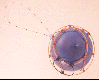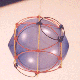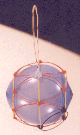The proton shell of 7 pins one radical string to a barrel shaped core. I'm pretty sure there is one or more spherical solutions, but I have been unable to solve for them. (Spherical solutions are very difficult to model with the inflexible gluing techniques used to construct these wire-frame examples. Once a wire is glued in place, it is difficult to remove and/or reposition it. Computer modeling techniques will remove that difficulty.)

## Stereograms of P7-41P Shell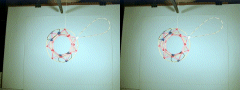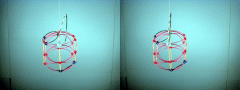Required Snap Points: 70 Available Snap Points: 69 Pinned Vector Bosons: 1 L1 = 31.7831937" (Structural) L2 = 40.6531901" (Equatorial) L3 = 28.1326453" (Filler) L4 = 18.7995816" (Polar) Model Diameter: 12.94031231" Loop Ratios: (1:2): 0.781813031 (1:3): 1.129762006 (1:4): 1.690633038 (2:3): 1.445053947 (2:4): 2.162451854 (3:4): 1.496450605 Loop Equation: 7(L1) + 1(L2) + 2(L3) + 2(L4) = 357" Snap Point Equation: 7(6) + 1(7) + 2(7) + 2(3) = 69 A.S.P. Radical Strings:+ 1 P.V.B. = 70 R.S.P.

# Proton 7: 3rd Degree, Polar Star (P7-30PS)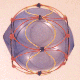This proton shell of 7 is a third degree sinusoidal solution with no pinned strings. Because there are an odd number of facets on this shell (7), the sinusoidal string wraps twice around the equator before closing on itself.

## Stereograms of P7-30PS Shell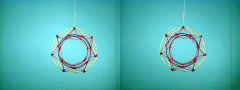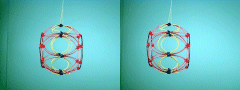Required Snap Points: 70 Available Snap Points: 70 Pinned Vector Bosons: 0 L1 = 28.21000867" (Structural) L2 = 24.96986844" (Polar) L3 = 109.5902024" (Sinusoidal) Model Diameter: 11.48551418" Loop Ratios: (1:2): 1.129762006 (1:3): 0.257413601 (2:3): 0.227847635 Loop Equation: 7(L1) + 2(L2) + 1(L3) = 357" Snap Point Equation: 7(6) + 2(7) + 1(14) = 70 R.S.P.

Copyright 1997 by Arnold J. Barzydlo
Go Back